Back to Kemp Acoustics HomeNext: Numerical implementation issues Up: Acoustic pulse reflectometry Previous: Input impedance from the   Contents

# Layer peeling bore reconstruction

We now want to analyse the input impulse response to find out the reflection coefficients (and therefore the changes in cross-section) at each step along the bore. This will enable us to reconstruct the bore profile. Bore reconstruction was first performed using the algorithm developed by Ware and Aki  as reviewed in . Since losses cannot be incorporated in the Ware Aki algorithm, we will instead use the lossy reconstruction method developed by Amir et al.  as used by Sharp [6,19,20].

Consider a junction between two infinite tubes. If a pressure wave is incident from negative infinity and no wave is incident from positive infinity, then the ratio of the backward and forward going waves is given by the reflection coefficient of equation (2.20).

Going back to the general case of an object attached to the source tube of a reflectometer from figure 5.1, at the first time step (ie. when the forward going pulse arrives at the input plane), there cannot be any backward going waves on surface 2. The ratio of the first term in the reflection sequence (or input impulse response) and the first term in the input sequence (which is unity) is therefore equal to the reflection coefficient from equation (2.20). We will label this reflection coefficient as
. The subscripts indicate that this reflection coefficient is for reflection from the discontinuity between surfaces 1 and 2 when waves are incident from the surface 1 side only.(5.8)
(5.9)

where we have labelled the surface area of surface 1 as
and the surface area of surface 2 as
. Nowfollows fromandby rearranging equation (5.8):
(5.10)

Now we know the cross-section on section 2, we will proceed to calculate the forward and backward going time sequences there. The forward going wave on section 2 is equal to the sum of the transmission of the forward going wave on section 1 and reflection of the backward going wave on section 2. Similarly, the backward going wave on section 1 is equal to the sum of the transmission of the backward going wave on section 2 and the reflection of the forward going wave on section 1. It can be expressed in matrix notation as follows: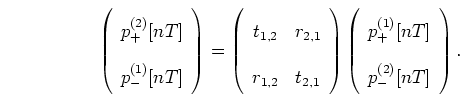(5.11)
gives the reflection coefficient from the discontinuity between surfaces 2 and 1 when waves are incident from the surface 2 side only:
(5.12)
gives the transmission coefficient from the discontinuity between surfaces 1 and 2 when waves are incident from the surface 1 side only. From equation (2.21) this is
(5.13)
gives the transmission coefficient from the discontinuity between surfaces 2 and 1 when waves are incident from the surface 2 side only:
(5.14)

We may rearrange the simultaneous equations in equation (5.11) to give the travelling waves on the right of the discontinuity in terms of the travelling waves on the left of the discontinuity: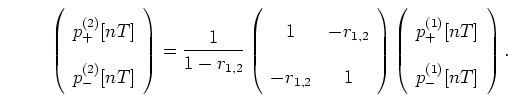(5.15)

This equation is performed for all values offromto
. For the current situation, the forward going sequence on surface 1 is an impulse,
, and the backward going pressure sequence on surface 1 is the input impulse response,. Notice that(5.16)

as no reflections return from surface 3 until.

The forward travelling pressure sequence, at the right hand side of the cylindrical section, will be found simply by adding a delay time of
to the forward going wave on the left, to account for the time taken to travel a distance of
.(5.17)is therefore known at
and in steps of
up to
.

The backward travelling wave at surface 3 is found by subtracting a delay of
from the backward travelling wave at surface 2.(5.18)
is therefore known atand in steps ofup to
. Notice that there is no backward going wave on surface 3 until:(5.19)

since no waves reach surface 3 until this time.

The ratio of the backward and forward travelling waves at surface 3, at time, is equal to the reflection coefficient
since this corresponds to a primary reflection.(5.20)

Subsections
Back to Kemp Acoustics HomeNext: Numerical implementation issues Up: Acoustic pulse reflectometry Previous: Input impedance from the   Contents
Jonathan Kemp 2003-03-24